# Functional Analysis

Also found in: Acronyms, Wikipedia.

## functional analysis

[¦′fəŋk·shən·əl ə′nal·ə·səs]
(mathematics)
A branch of analysis which studies the properties of mappings of classes of functions from one topological vector space to another.
(systems engineering)
A part of the design process that addresses the activities that a system, software, or organization must perform to achieve its desired outputs, that is, the transformations necessary to turn available inputs into the desired outputs.
McGraw-Hill Dictionary of Scientific & Technical Terms, 6E, Copyright © 2003 by The McGraw-Hill Companies, Inc.
The following article is from The Great Soviet Encyclopedia (1979). It might be outdated or ideologically biased.

## Functional Analysis

an aggregate of chemical and physical methods of analysis, primarily for organic substances, that are based on the determination of reactive groups of atoms (individual atoms)—functional groups—in molecules. Examples of such groups are the hydroxyl (—OH), carboxyl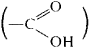nitro (—NO2), and amino (—NH2) groups.

Functional analysis is used to confirm the presumed structure of a substance or reaction mechanism and to establish the percent content of individual compounds of known structure in a mixture. The chemical methods include the use of characteristic reactions of the functional groups, such as the reduction of a nitro group to an amino group, which is easily identified; and the formation of a stained complex upon the interaction of alcohols with ammonium hexanitratocerate, or eerie ammonium nitrate: ROH + (NH4)2Ce(N03)6 → (NH4)2Ce(OR)(N03)5 + HN03.

Many functional groups can also be detected and quantitatively determined by means of nuclear magnetic resonance, mass spectrometry, and infrared spectroscopy. For example, special diagrams of infrared-emission absorption by functional groups (Colthup charts) are used to identify the groups, while absorption intensity is used to determine the quantitative content of the groups.

### REFERENCES

Bobrański, B. Kolichestvennyi analiz organicheskikh soedinenii. Moscow, 1961. (Translated from Polish.)
Terent’ev, A. P. Organicheskii analiz: Izbr. trudy. Moscow, 1966.
Cheronis, N. D., and T. S. Ma. Mikro- i polumikrometody organicheskogo funktsional’ nogo analiza. Moscow, 1973. (Translated from English.)
Klimova, V. A. Osnovnye mikromelody analiza organicheskikh soedinenii. Moscow, 1975.

IU. A. KLIACHKO

## Functional Analysis

a branch of modern mathematics whose principal concern is the study of infinite-dimensional spaces and their mappings. Linear spaces and mappings have been studied more extensively than others.

A characteristic of functional analysis is the combining of methods of classical analysis, topology, and algebra. By abstracting from particular instances it is possible to choose axioms and use them to construct a theory that includes classical problems as special cases and makes possible the solution of new problems. The process of abstraction is of independent value, since it clarifies various situations, permits us to dispense with what is superfluous, and uncovers unexpected connections. Abstraction thus enables us to obtain a deeper insight into the essence of mathematical concepts and to develop new paths of investigation.

The development of functional analysis paralleled the development of modern theoretical physics. In fact, the language of functional analysis best mirrors the laws of quantum mechanics and quantum field theory. In turn, these physical theories have had a substantial effect on the body of problems and methods of functional analysis.

Development. Functional analysis became an independent branch of mathematics in the late 19th and early 20th centuries. G. Canter’s set theory played an important role in the formulation of the general concepts of functional analysis. The development of set theory and of axiomatic geometry gave rise to metric topology and to the more general set-theoretic topology pioneered by M. Fréchet and F. Hausdorff. These disciplines study abstract spaces, that is, sets of arbitrary objects for which there is defined in some way the notion of proximity.

The abstract spaces of greatest importance for mathematical and functional analysis are spaces of functions, that is, spaces whose elements are functions; hence the term “functional analysis.” D. Hubert’s deep study of integral equations gave rise to the spaces l2 and L2(a, b). Generalizing these spaces, F. Riesz investigated the spaces lp and Lp(a, b), and in 1922, S. Banach introduced complete normed linear spaces, or Banach spaces. In the 1930’s and 1940’s, T. Carleman, Riesz, and the American mathematicians M. Stone and John von Neumann constructed the abstract theory of self-adjoint operators in Hubert space.

In the Soviet Union the first papers dealing with functional analysis appeared in the 1930’s. Important contributions were made by A. N. Kolmogorov to the theory of linear topological spaces (1934), by N. N. Bogoliubov to invariant measures and dynamical systems (1936), and by L. V. Kantorovich and disciples to the theory of semiordered spaces and to applications of functional analysis to numerical methods (1937). M. G. Krein and disciples studied in depth the geometry of Banach spaces, convex sets and cones in such sets, the theory of operators, and connections with various problems of classical mathematical analysis (1938). I. M. Gel’fand and disciples investigated the theory of normed rings, or Banach algebras (1940).

The present stage of the development of functional analysis is characterized by a strengthening of ties with theoretical physics and with such branches of classical analysis and algebra as the theory of functions of many complex variables and the theory of partial differential equations.

The concept of a space. The most general spaces investigated in functional analysis are linear topological spaces (topological vector spaces), that is, linear spaces X over the field of complex numbers C (or the field of real numbers IR) that are at the same time topological spaces and are such that the linear operations are continuous in the relevant topology.

A special but very important situation arises when it is possible to introduce for vectors in the linear space X a norm (length) whose properties are generalizations of the properties of the length of vectors in ordinary Euclidean space. Specifically, the norm of an element xX is a real number ‖x‖ such that ‖x‖ ≥ 0, with ‖x‖ = 0 if, and only if, x = 0; furthermore,

‖λx‖ = |λ| ‖x‖ λ ∈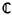‖x + y‖ ≤ ‖x‖ + ‖y‖

Such a space is called a normed linear space; its topology is defined by means of the metric d(x, y) = ‖x – y‖ (so that xnx if ‖xnx‖ → 0).

In many problems there arises the more special situation where it is possible to introduce in a linear space X an inner product, that is, a generalization of the ordinary inner product in Euclidean space. Specifically, the inner product of elements x, yX is a complex number (x, y) such that, at all times, (x, x) = 0 and only if, x = 0;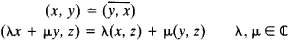Also,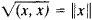is the norm of x. Such a space is called a pre-Hilbert space.

A crucial property of spaces in functional analysis is their completeness, which can be defined in the following way: if a sequence {xn} of elements in X has the property that ‖xn – xm → 0 as n, m →, ∞ then the sequence has a limit x,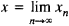and this limit is also an element of X. A complete normed linear space is called a Banach space, and a complete pre-Hilbert space is called a Hubert space. The process of completing a metric space is analogous to the transition from the rational to the real numbers and makes a normed linear space into a Banach space and a pre-Hilbert space into a Hilbert space.

Ordinary Euclidean space is one of the simplest examples of a (real) Hilbert space. In functional analysis, however, the main role is played by infinite-dimensional spaces, that is, spaces in which there are infinitely many linearly independent vectors. Of particular interest are such spaces whose elements are classes of complex-valued functions defined on some set T with the usual algebraic operations—that is, (x + y)(t) = x(t) + y(t) and (λ)(t) = λ(t). An example is the Banach space C(T) of all continuous functions, with T a compact subset of the n-dimensional space IR” and with norm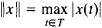Another example is the Banach space Lp (T) of p-integrable (p ≥ 1) functions on T with norm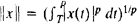. A third example is the Banach space lp of all sequences such that ∑i|xi|p < ∞, j ∈ ℤ (the set of integers), and with norm ‖x‖ = (∑|xi|p)1/p. For p = 2 the spaces l2 and L2(T) are Hilbert spaces; in L2(T), say, an inner product is given by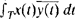. A fourth example is the linear topological space D(IR) of infinitely differentiable functions on IR with compact support [that is, vanishing outside some interval (a, b)]; here, xnx as n → ∞ provided that the xn (t) have uniform compact support [that is, (a, b) is independent of n] and the xn (T) and their derivatives converge uniformly to x(t) and its derivatives, respectively.

All the above examples are infinite-dimensional spaces. This fact is most easily seen in l2: the vectors e = {0, . . ., 0, 1, 0, . . .} are linearly independent.

From a geometric point of view the simplest spaces are Hubert spaces H, whose properties are closest to those of finite-dimensional Euclidean spaces En. In particular, two vectors x, yH are said to be orthogonal (x – y) if (x, y) = 0. Every xH has a projection to an arbitrary subspace F (a subspace F of H is a closed linear subset of H), that is, a vector xf such that xxF– f for every f ∈ F. As a result of this fact, many geometric constructions intrinsic to En can be carried over from En. to H and often have analytic significance in H. For example, the usual orthogo-nalization procedure enables us to conclude that H contains an orthonormal basis—that is, a sequence of vectors ejjZ, in H such that ‖ej‖ = 1, ej ≠ ek for j ≠ k, and for every xH we have the “coordinate” expansionwhere xj-= (x, ej) and ‖x‖2 = ∑|xJ|2 (for the sake of simplicity we assume that H is separable, that is, H contains a countable everywhere dense subset). If we take H to be L2 (0, 2π) and put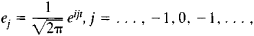then (1) yields the Fourier expansion of x(t) ∈ L2 (0, 2π); this expansion converges to x(t) in the mean. Also, (1) shows that the correspondence between H and I2 ∋ {xj}, j, is an isomorphism, that is, a linear isometry. Thus, in the above context, /2 is a universal space.

Geometric issues of the kind considered above become far more complex when we go from Hilbert to Banach spaces and, especially, to topological vector spaces, owing to the nonexistence in these spaces of orthogonal projections. Consider, for example, the basis of lp in the sense that the expansion (1) is valid. In most known examples of Banach spaces it has been possible to construct bases, but it was not until 1972 that the problem (stated by Banach and J. Schauder more than 50 years earlier) of the existence of a basis in every separable Banach space was solved in the negative. Similar “geometric” themes, concerned with the elucidation of the properties of convex, compact, and other sets in, for example, Banach spaces, play an important role in functional analysis, and easily formulated questions often have altogether nontrivial solutions. These themes are closely associated with the question of isomorphism of spaces and with the search for universal representatives in some class of spaces (in the sense in which l2 is universal in the class of Hilbert spaces).

A large part of functional analysis is devoted to the detailed study of particular spaces, since their properties usually determine the nature of the solution of a problem obtained by methods of functional analysis. A typical example is furnished by the imbedding theorems for Sobolev spaces and generalizations thereof. The simplest space of this kind is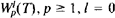, 1, 2, . . ., defined as the completion of the infinitely differentiable functions x(t) on T with respect to the norm ∑‖Dαx‖ in Lp(T), where the sum is taken over all derivatives Dα of order ≤l. The imbedding theorems clarify the degree of smoothness of the elements of the space obtained by the completion process.

The needs of mathematical physics have given rise to a large number of concrete spaces obtained from known ones by means of specific constructions. Some of the most important of these constructions are the following:

(a) The orthogonal sum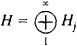of Hilbert spaces Hj. This construction is similar to the process, described by formula (1), of forming H out of one-dimensional spaces.

(b) Factorization and completion. Starting with a vector space X, we define on it a quasi-inner product [that is, we admit the possibility (x, x) = 0 for x ≠ 0], at times of a rather exotic nature, and construct H by completing X with respect to (. . .) after the preliminary step of identifying with 0 all vectors x for which (x, x) = 0.

(c) The tensor productIts formation is analogous to the transition from functions f(x1) of one variable to functions f(x1 . . ., xq) of many variables.

(d) The projective limitof Banach spaces. Here, roughly speaking, xn as n → ∞ if ‖xn – x‖α 0 as n → ∞ for every α.

(e) The inductive limit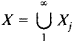of Banach spaces X1 ⊂ X2 . . . . Here, xn → x as n → ∞ if all x1, from some J0 on, belong to the same xj0 and if in it ‖xn – x‖ – 0 as n → ∞.

The last two constructions are commonly used to construct topological vector spaces. A relevant example is that of a kernel space—that is, the projective limit of Hubert spaces Hα such that for every α there is a β with Hα ⊂ Hα and this inclusion is a Hil-bert-Schmidt imbedding. The space D(IR) is an example of a kernel space.

A great deal is known about the branch of functional analysis concerned with spaces with conical structure x > 0 (semiordered spaces). A example of such a space is real C(T), where x > 0 if x(t) ≥ 0 for all t ∈ T.

Operators (general concepts); functionals. Let X and Y be linear spaces. A mapping A: Xy is said to be linear if for all x, y ∈ X, λ, μ ∈,

A(λx + μy) = λAx + μAy

A linear mapping is usually called a linear operator. In the case of finite-dimensional X, Y the structure of a linear operator is simple. If x1 . . ., xn are the coordinates of a vector x relative to a basis of X and (Ax)1, . . ., (Ax)n are the coordinates of Ax relative to a basis of Y, then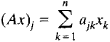Upon transition to infinite-dimensional topological vector spaces, matters become far more complicated. In such spaces we must, first of all, distinguish between continuous and discontinuous linear operators (linear operators on finite-dimensional spaces are always continuous). Thus, if X = Y = L2 (a, b) and A:X → Y is defined by the equation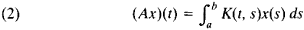where K(t, s)—a bounded function—is the kernel of A, then A is continuous. On the other hand, the differentiation operator defined on the subspace C1(a, b) ⊂ L2 (a, b) by means of the equation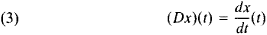is discontinuous. In general, a characteristic feature of discontinuous operators is that they are not defined on the whole space.

For a continuous operator A: X → Y, where X and Y are Banach spaces,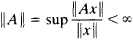This fact explains why such operators are called bounded. The set of all bounded operators ℒ(X, Y) with the usual algebraic operations form a Banach space with norm ‖A‖. To a large extent, the properties of ℒ(X, Y) mirror the properties of X and Y, especially if Y is one-dimensional, that is, for linear continuous mappings l:X→, known as functionals. The space ’ℒ(X,) is called the adjoint of A” and is denoted by X’.

If X = H is a Hilbert space, then the structure of H’ is simple: just as in the finite-dimensional case, every functional l(x) has the form (x, a), where a is a vector in H that depends on l (Riesz’ theorem). The correspondence H’ → H is an isomorphism between the two spaces, and we may say that H’ = H. In the case of a general Banach space X the situation is far more complex: the spaces X, X’ = (X’)’, ... may turn out to be distinct.

In general, the question of the existence of nontrivial (that is, different from 0) functional on Banach spaces is not a simple one. According to the Hahn-Banach theorem, if F is a subspace of X that does not reduce to a single point and if there exists lF’, then this functional can be extended to X —that is, to a functional in X’ —without change of norm. For lX the equation l(x) = c defines a hyperplane, the translate of X by some vector, of dimension one less than X. Thus, results of the type illustrated by this theorem admit of simple geometric interpretations.

There is a specific sense in which the space X’ is “better” than X. Thus, in addition to a norm, we can introduce in X” the weak topology [roughly speaking, ln → l as n → ∞ if lnx) → l(x) for every xX], in which a sphere, that is, the set of all xX with |x| ≤ r, is compact (no such effect is ever true in an infinite-dimensional space in the topology based on the norm). We consequently can study in greater detail a number of geometric questions for sets in X’. For example, the Krein-Milman theorem asserts that every compact convex set in X’ is the closed hull of its extreme points.

An important problem of functional analysis is to find the general form of functionals on a specific space. In many cases, in addition to the case of a Hubert space, such a general form has been found. For example, (lp)’, p > 1, consists of functions of the form πxjāj, where {aj} ∈ lq, (1/p) + (1/q) = 1. However, in the case of most Banach spaces, and especially in the case of topological vector spaces, the corresponding functionals are not readily constructed by methods of classical analysis. Thus, for fixed t0 and m there is defined on D(IR) the functional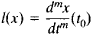For m = 0 this functional can be expressed in a “classical” manner—that is, by means of an integral. For m ≥ 1, however, a classical representation is no longer possible.

The elements of (D(IR))’ are called generalized functions, or distributions. If we regard distributions as elements of an adjoint space, then we can also construct them when D(IR) is replaced by a space 0 of functions that are differentiable an infinite or a finite number of times; here, an important role is played by the triple of spaces Φ H ⊃ Φ, where H is the initial Hubert space and Φ is a topological vector space (possibly a Hubert space with different inner product), such as the space.

The differential operator D in (3) is continuous if it is viewed as mapping into L2[a, b] from the space C1[a b] with norm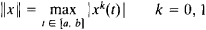In many problems, particularly in spectral theory, however, it is necessary to interpret such differential operators as acting in just one space. These and related problems have given rise to a general theory of unbounded and, in particular, unbounded self-adjoint and Hermitian operators.

Special classes of operators; spectral theory. In many problems it is indispensable to study the solvability of equations of the form Cx = y, where C is a certain operator, y ∈ Y a given vector, and xX is a required solution vector. For example, if X = Y = L2(a, b) and C = E – A, where A is the operator in (2) and E is the identity operator, then we obtain a Fredholm integral equation of the second kind, and if C is a differential operator, then we obtain a differential equation.

Unless we restrict ourselves to suitable classes of operators we cannot expect to obtain results that bear sufficient resemblance to relevant results of linear algebra. One of the most important classes of operators closest to the finite-dimensional case is the class of compact, or completely continuous, operators, which are characterized by the fact that they map bounded sets in X onto sets in y with compact closure; A in (2) is an example of such an operator. Riesz constructed for completely continuous operators a theory of solvability of x – Ax = y that is fully analogous to the finite-dimensional case; in particular, this theory includes the theory of the above-mentioned integral equations.

In a variety of problems of mathematical physics the eigenvalue problem arises: given an operator A:X → X, we are required to find out if there exist solutions φ ≠ 0 (eigenvectors) of the equation = λφ for some λ ∈ C (the corresponding eigenvalue). The action of A on Φ is particularly simple, since it amounts to multiplication by a scalar. This fact has useful consequences. If, for example, the eigenvectors ej, j ∈ ℤ, of an operator A form a basis of the space X, that is, if we have an expansion of type (1), then the action of A can be presented in a manner that has a strong intuitive appeal: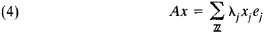where λj is the eigenvalue corresponding to xj. For a finite-dimensional case we know precisely under what conditions such a representation holds. If there are multiple eigenvalues, then, in general, there are not enough eigenvectors to form a basis in X, and it is necessary to supplement the eigenvectors with associated vectors. The set Sp A of eigenvalues of A is called the spectrum of A

The extension of these ideas to infinite-dimensional spaces was first given by Hilbert for such integral operators as A in (2) with symmetric kernel, that is, K(t, s) = K(s, t) and is real. A similar theory was then developed for compact self-adjoint operators in Hilbert space. Efforts to extend the theory to very simple non-compact operators uncovered difficulties due to the very definition of the spectrum. For example, the bounded operator on L2[a, b] given by

(5) (Tx)(t) = tx(t)

has no eigenvalues. Consequently, the spectrum concept was revised and generalized

The present-day definition of “spectrum” follows. Let A” be a Banach space, and let Aℒ(X, X). A pointis said to be regular for A if the inverse operator (A – zE)–l = Rz (that is, the inverse mapping) exists and belongs to ℒ(X, X). The spectrum Sp A of A is the complement of the set of regular points of A. Just as in the finite-dimensional case, the spectrum of A is not empty and is located in the circle ‖z‖ ≤ ‖A‖.

By means of these concepts it was possible to construct a theory of operators—that is, to give reasonable definitions of certain functions of operators. For example, if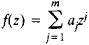is a polynomial, then(the power of an operator is understood as the result of multiplying the operator by itself a suitable number of times). If, however, f(z) is an analytic function, then it is not always possible to interpret f(A) in such a simple manner. In fact, if/(z) is analytic in a neighborhood of the spectrum of A, if Γ is a contour containing the spectrum oí A, and if Γ is contained in the domain of ana-lyticity of f(z), then we putHere, the algebraic operations on functions go over into analogous operations on operators; that is, the mapping f(z) → f(A) is a homomorphism. By using these constructions we cannot settle such questions as the completeness of the set of eigenvectors and associated vectors for all operators. For self-adjoint operators, however, which are of greatest interest in, for example, quantum mechanics, a corresponding theory has been completely worked out.

Let H be a Hubert space. A bounded operator A:HH is called self-adjoint if (Ax, y) = (x, Ay) (for unbounded A the definition is more complicated). If H is n-dimensional, then it contains an orthonormal basis of eigenvectors of the self-adjoint operator A; that is, we have the expansions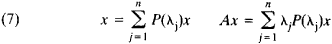where P(λj) is the projection operator to the subspace spanned by the eigenvectors of A corresponding to the eigenvalue λj.

It turns out that these formulas can be extended to arbitrary self-adjoint operators on H except that the existence of the projection operators P(λj) depends on the existence of eigenvectors [it may be recalled that the operator T in (5) has no eigenvectors]. The sums in (7) are now replaced by Stieltjes integrals of a nondecreasing operator-valued function E(λ), which equalsin the finite-dimensional case; E(λ) is called the resolution of unity, or spectral measure, and increases at just the points of the spectrum of A.

Formulas of type (7) hold for generalized functions. Specifically, the relations (7) hold for a triple of spaces Φ’ ⊃ H ⊃ Φ, where Φ is, say, a space with a kernel and A maps Φ into Φ’ and is continuous, except that sums go over into integrals with respect to a certain scalar measure and E(λ) now “projects” Φ into Φ’, yielding vectors in Φ that are eigenvectors, in a generalized sense, of A corresponding to the eigenvalue X. Similar results hold for normal operators, that is, operators that commute with their ad-joints. An example is presented by unitary operators U, that is, finite operators that map H onto H and preserve inner products. The spectra of such operators lie on the circle |z| = 1, which is the path of integration in analogues of formulas (6). (see alsoSPECTRAL ANALYSIS OF LINEAR OPERATORS.)

Nonlinear functional analysis. The development and refinement of the concept of a space was accompanied by the development and generalization of the concept of a function. Finally, it became necessary to consider possibly nonlinear mappings of one space into another (often into the initial space). One of the central problems of nonlinear functional analysis is the study of such mappings. Just as in the linear case, mappings into C or IR are called functionals. For nonlinear mappings (in particular, nonlinear functionals) we can define in various ways such concepts as a differential and a directional derivative so as to be similar to the corresponding concepts of classical analysis. If we single out in the mappings quadratic and higher-degree terms, then we are led to an analogue of Taylor’s formula.

An important problem of nonlinear functional analysis is the problem of fixed points of mappings, where x is said to be a fixed point of F if Fx = x. Many problems of the solvability of operator equations, as well as problems of finding eigenvalues and eigenvectors of nonlinear operators, reduce to fixed-point problems. In solving equations involving nonlinear operators we encounter branch points of solutions—a phenomenon essential for nonlinear functional analysis.

The study of fixed points and branch points makes use of topological methods and concepts. An example of such a method is the extension to infinite-dimensional spaces of L. Brouwer’s theorem on the existence of fixed points of mappings of finite-dimensional spaces. An example of the topological concepts used is the degree of a mapping. Contributors to the development of the topological methods of functional analysis include the Polish mathematician J. Schauder, the French mathematician J. Leray, and the Soviet mathematicians M. A. Krasnosel’skii and L. A. Liusternik.

Banach algebras; representation theory. The only problems investigated during the early stages of the development of functional analysis were problems whose formulation and solution required only the performance of linear operations on the elements of a space. Perhaps the sole exceptions to this statement are the theory of rings of operators (J. von Neumann, 1929) and the theory of absolutely convergent Fourier series (N. Wiener, 1936).

The late thirties saw the appearance of papers dealing with the theory of normed rings (now referred to as Banach algebras) by such mathematicians as the Japanese M. Nagumo and the Soviets I. M. Gel’fand, G. E. Shilov, and M. A. Naimark. In this theory one axiomatizes not only vector space operations but also the operation of multiplication, it being stipulated that ‖xy‖ ≤ ‖x‖ ‖y‖. Typical Banach algebras are rings of bounded operators on a Banach space (with multiplication defined as composition in stated order) and various function spaces, such as C(T) with the usual operation of multiplication, L,(IR) with convolution as multiplication, and the broad generalization of these spaces that is the class of group algebras (of a topological group G) consisting of complex-valued functions or measures defined on G with (not necessarily equivalent variants of) convolution as multiplication.

Let થ be a commutative Banach algebra with unity—that is, xy = yx for all x, y ∈ થ, and the algebra contains an element e such that ex = xe = x, ‖e‖ = 1. An ideal of થ is a subspace I ⊂ થ such that, for all x ∈ થ and a ∈ I, xa is in I. An ideal is maximal if it is not contained in a nontrivial (that is, different from થ) ideal. It is possible to define on the set M of maximal ideals a compact topology in such a way that to every x ∈ થ there corresponds a complex-valued continuous function x̂(t) on M that preserves sums and products [that is, to x + y and xy there correspond x̂(t) + ŷ(t) and x̂(t)ŷ(t), respectively]. In other words, as Gel’fand’s theorem asserts, there exists a homomorphism from થ into C(M).

In the noncommutative case, the most extensively studied algebras are Banach algebras with involution, that is, a mapping *: થ → થ such that for all x, y ∈, થ, λ,(x*)*y = x

(λx + µy)* = λx* + µ̄y*

(xy)* =y*x*

A relevant example is the Banach algebra ℒ(H) of bounded operators on a Hilbert space H with involution defined as transition to the adjoint operator. [A* is the adjoint of A ∈ ℒ(H) if (A *x, y) = (x, Ay) for any x, yH; the corresponding definition for an unbounded operator is more complicated.] In particular, a self-adjoint operator is characterized by the property A* = A and a unitary operator by the property U* = U–l. Let થ be a Banach algebra with involution such that ‖xx*‖ = ‖x2 for all x ∈ થ (that is, let થ be a C*-algebra). The Gel’fand-Naimark theorem states that થ is isometrically isomorphic to a subalgebra of the algebra ℒ(H). Moreover, in the commutative case થ is isomorphic to C(M).

The multiplicative structure of the Banach algebra ℒ(H) plays an important role in the representation theory of groups and algebras. In general, the representation of abstract mathematical objects by simpler or, at least, more common objects is a powerful mathematical technique. For example, the spectral resolution (7) of a self-adjoint operator A may be interpreted as the representation of A in the form of an integral of operators of multiplication by the independent variable λ of measurable functions in a certain class: A = ʃλdE(λ) . If we consider multiplication of functions in that class by Borel functions, then we obtain a representation of the commutative ring of operators on Hilbert space. More general examples are given below.

The most advanced theory is that of linear representations of topological groups (including finite topological goups). A linear representation of a topological group G is a homomorphism π:G → ℒ(X), where ℒ(X) is a group (under multiplication) of linear operators on a topological vector space X —in other words, ℒ(X) is essentially a group of mappings of X. Continuous representations are usually considered—that is, representations such that the mapping {g, x}π(g)x is continuous for all gG, xX. There are similar definitions for representations of a ring and an algebra, in particular, a Banach algebra; here, the linear structure of થ is required to correspond to the linear structure of the ring ℒ(X). The class of unitary representations is best known; it is the most important class of representations from the point of view of applications to dynamical systems, quantum mechanics, and quantum field theory. Here, X = H is a Hilbert space, and π(g) are unitary operators for all gG.

Let G be a locally compact group, and let dg be a Haar measure on G, that is, a right-invariant measure on the ring ℬ of Borel subsets of G: for all B ∈ ℬ and hG we have ʃBh dg = ʃB dg. Further, let L1(G, dg) be the group algebra of functions on G integrable with respect to dg. To every hG there corresponds a unitary (owing to the invariance of dg) operator Th of group translation defined on L1(G, dg). Specifically, for f(g) ∈ L1(G, dg), g ∈ G, we have Thf(g) = f(gh), with Th1Th2 = Thh1h2. (Th)–1 = Th–1, that is, the mapping π(h) = Th is a unitary representation of G. In turn, the group of translations (the image of G under π) can be mapped homomorphically onto the space L1(G, dg), which may thus be viewed as the realm of action of the inverse representation of the operators Th by functions f(g).

If G is commutative, then the structure of translation operators is given by the formula

ʃG f(hg–1) k(g) d(g) = ʃG X(h)F(X)K(X)dX

Here, X(h) is a character of the group G, that is, a continuous function on G with |X(h)| = 1 and X(h1h2) = X(h1)X(h2); in addition, dX is a Haar measure on the group of characters G, and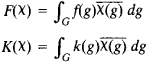is the generalized Fourier transform of the functions f(g) and k(g), which is continued to an isomorphism of L2 (G, dg) into L2 (G, dX). For noncommutative groups the situation is much more complicated. If G is compact then the structure of the group of translation operators (or, more concisely, the group of translations) is easy to describe; in that case L2 (G, dg) is the direct sum of finite-dimensional, translation-invariant subspaces. If G is not compact, then L2 (G, dg) can also be broken down into simpler invariant parts, the direct sum being replaced by a direct integral.

If G = IR, then the theory of unitary representations can be reduced to the theory of self-adjoint operators. Stone’s theorem states that a one-parameter group of unitary operators Tλ, λ ∈ IR, on a Hubert space H admits of the representation Tλ = exp , where A is a self-adjoint operator; the operator A is called the infinitesimal generator of the group {Tλ}. This result has important applications in the study of transformations of phase space in classical mechanics. This connection with mechanics and its application to certain problems in statistical mechanics are the foundation of the extensive branch of functional analysis known as er-godic theory.

The connection between one-parameter groups of transformations and their generators can be considerably generalized. Instead of being unitary, the operators Tλ may act on Banach and even more general spaces, and the values of λ may be restricted to the ray λ ≥ 0. The resulting theory of semigroups of operators has applications to the theory of partial differential equations and the theory of the stochastic processes known as Markov processes.

### REFERENCES

Liusternik, L. A., and V. I. Sobolev. Elementy funktsional’nogo analiza, 2nd ed. Moscow, 1965.
Kolmogorov, A. N., and S. V. Fomin. Elementy teorii funktsii i funktsional’nogo analiza, 4th ed. Moscow, 1976.
Akhiezer, N. I., and I. M. Glazman. Teorii lineinykh operatorov v gil’bertovomprostranstve, 2nd ed. Moscow, 1966.
Vulikh, B. Z. Vvedenie v teoriiu poluuporiadochennykh prostranstv. Moscow, 1961.
Banach, S. S. Kurs funktsional’nogo analizu. Kiev, 1948.
Riesz, F., and B. Szőkefalvi-Nagy. Lektsii po funktsional’nomu analizu. Moscow, 1954. (Translated from French).
Sobolev, S. L. Nekotorye primeneniia funktsional’ nogo analiza v matematicheskoi fizike. Leningrad, 1950.
Kantorovich, L. V., and G. P. Akilov. Funktsional’nyi analiz v normirovannykh prostranstvakh. Moscow, 1959.
Kransnosel’skii, M. A., and P. P. Zabreiko. Geometricheskiemetody nelineinogo analiza. Moscow, 1975.
Naimark, M. A. Normirovannye kol’tsa, 2nd ed. Moscow, 1968.
Rudin, W. Funktsional’nyi analiz. Moscow, 1975. (Translated from English.)
Yosida, K. Funktsional’nyi analiz. Moscow, 1967. (Translated from English.)
Dunford, N., and J. Schwartz. Lineinye operatory, parts 1–3. Moscow, 1962–74. (Translated from English.)
Hille, E., and R. Phillips. Funktsional’nyi analiz i polugruppy, 2nd ed. Moscow, 1962. (Translated from English.)
Edwards, R. E. Funktsional’nyi analiz: Teoriia i prilozheniia. Moscow, 1969. (Translated from English.)

IU. M. BEREZANSKII and B. M. LEVITAN

References in periodicals archive ?
In this way the functional analysis will help to the clearly defining of the functional requirements.
Within the literature, results of analogue functional analysis, have generally shown that SIB occurs with higher probability in those conditions in which access to social attention and tangible or food items are contingent upon SIB (Barrera, Violo & Graver, 2007; Iwata, Pace et al., 1994; Oliver, Hall & Murphy, 2005; Repp, Felce & Barton, 1988; Richman & Lindauer, 2005; Symons, Hoch, Dahl & McComas, 2003).
Analogue functional analysis is a type of assessment conducted to determine the function of behaviors of interest.
The observations served to corroborate the researchers' initial hypothesis statements and inform their design of a functional analysis.
This, too, has always been a possibility under historical analytical and reporting principles, but in practice may be more likely in view of the emphasis now placed on a functional analysis of a taxpayer's transactions in their economic context.
Applied Nonlinear Functional Analysis: An Introduction
(2001) used a multielement design to evaluate noise as an establishing operation for challenging behavior, and then incorporated noise into a functional analysis in which there was a specific noise condition, a play condition, and a no interaction condition.
We identified four main areas of further discussion: the reticulation strategy, the importance of functional analysis, the implication of learning history in clinical work, and the development of research in RFT for clinical applications.
of Malaysia, Kuala Lumpur) present methods of nonlinear functional analysis and their application to neuroscience.
While the level of math discussed in the text is what a graduate student would encounter after studying functional analysis, all of the underlying tools of functional analysis necessary to work through the text are included, chapter by chapter, as they arise.
In addition, these fears may also understandably make staff less likely to use assessment procedures that would increase the likelihood of the challenging behavior (e.g., functional analysis), even though such environmental manipulations have the most empirical support for their use.

Site: Follow: Share:
Open / Close